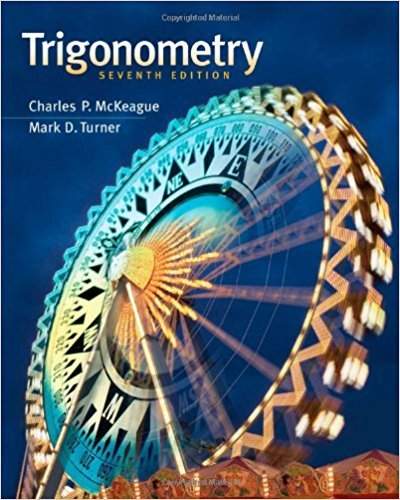×
×

# Solutions for Chapter 1-10: Complex Numbers and Polar Coordinates## Full solutions for Trigonometry | 7th Edition

ISBN: 9781111826857Solutions for Chapter 1-10: Complex Numbers and Polar Coordinates

Solutions for Chapter 1-10
4 5 0 341 Reviews
29
5
##### ISBN: 9781111826857

Trigonometry was written by and is associated to the ISBN: 9781111826857. Since 1 problems in chapter 1-10: Complex Numbers and Polar Coordinates have been answered, more than 25770 students have viewed full step-by-step solutions from this chapter. This expansive textbook survival guide covers the following chapters and their solutions. This textbook survival guide was created for the textbook: Trigonometry, edition: 7. Chapter 1-10: Complex Numbers and Polar Coordinates includes 1 full step-by-step solutions.

Key Math Terms and definitions covered in this textbook
• Cofactor Cij.

Remove row i and column j; multiply the determinant by (-I)i + j •

• Condition number

cond(A) = c(A) = IIAIlIIA-III = amaxlamin. In Ax = b, the relative change Ilox III Ilx II is less than cond(A) times the relative change Ilob III lib II· Condition numbers measure the sensitivity of the output to change in the input.

• Elimination.

A sequence of row operations that reduces A to an upper triangular U or to the reduced form R = rref(A). Then A = LU with multipliers eO in L, or P A = L U with row exchanges in P, or E A = R with an invertible E.

• Fourier matrix F.

Entries Fjk = e21Cijk/n give orthogonal columns FT F = nI. Then y = Fe is the (inverse) Discrete Fourier Transform Y j = L cke21Cijk/n.

• Hermitian matrix A H = AT = A.

Complex analog a j i = aU of a symmetric matrix.

• Identity matrix I (or In).

Diagonal entries = 1, off-diagonal entries = 0.

• Independent vectors VI, .. " vk.

No combination cl VI + ... + qVk = zero vector unless all ci = O. If the v's are the columns of A, the only solution to Ax = 0 is x = o.

• Left nullspace N (AT).

Nullspace of AT = "left nullspace" of A because y T A = OT.

• Markov matrix M.

All mij > 0 and each column sum is 1. Largest eigenvalue A = 1. If mij > 0, the columns of Mk approach the steady state eigenvector M s = s > O.

• Multiplicities AM and G M.

The algebraic multiplicity A M of A is the number of times A appears as a root of det(A - AI) = O. The geometric multiplicity GM is the number of independent eigenvectors for A (= dimension of the eigenspace).

• Normal equation AT Ax = ATb.

Gives the least squares solution to Ax = b if A has full rank n (independent columns). The equation says that (columns of A)·(b - Ax) = o.

• Orthonormal vectors q 1 , ... , q n·

Dot products are q T q j = 0 if i =1= j and q T q i = 1. The matrix Q with these orthonormal columns has Q T Q = I. If m = n then Q T = Q -1 and q 1 ' ... , q n is an orthonormal basis for Rn : every v = L (v T q j )q j •

• Polar decomposition A = Q H.

Orthogonal Q times positive (semi)definite H.

• Projection matrix P onto subspace S.

Projection p = P b is the closest point to b in S, error e = b - Pb is perpendicularto S. p 2 = P = pT, eigenvalues are 1 or 0, eigenvectors are in S or S...L. If columns of A = basis for S then P = A (AT A) -1 AT.

• Row space C (AT) = all combinations of rows of A.

Column vectors by convention.

• Special solutions to As = O.

One free variable is Si = 1, other free variables = o.

• Spectrum of A = the set of eigenvalues {A I, ... , An}.

Spectral radius = max of IAi I.

• Standard basis for Rn.

Columns of n by n identity matrix (written i ,j ,k in R3).

• Subspace S of V.

Any vector space inside V, including V and Z = {zero vector only}.

• Transpose matrix AT.

Entries AL = Ajj. AT is n by In, AT A is square, symmetric, positive semidefinite. The transposes of AB and A-I are BT AT and (AT)-I.

×